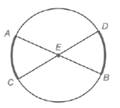Chapter 6.1, Problem 30E### Elementary Geometry for College St...

6th Edition
Daniel C. Alexander + 1 other
ISBN: 9781285195698

#### Solutions

Chapter
Section### Elementary Geometry for College St...

6th Edition
Daniel C. Alexander + 1 other
ISBN: 9781285195698
Textbook Problem
1 views

# In Exercises 30 and 31, complete each proof. Given: Diameters A B ¯ and C D ¯ in ⊙ E Prove: A C ⌢ ≅ D B ⌢PROOF Statements Reasons 1. ? 1. Given 2. ∠ A E C ≅ ∠ D E B 2. ? 3. m ∠ A E C = m ∠ D E B 3. ? 4. m ∠ A E C = m A C ⌢ and m ∠ D E B = m D B ⌢ 4. ? 5. m A C ⌢ = m D B ⌢ 5. ? 6. ? 6. If two arcs of a circle have the same measure, they are ≅

To determine

To prove: The ACDB by using the provided figure.

Explanation

Given: The diameters AB¯ and CD¯ in E

Postulate used:

Central angle postulate:

In a circle, the degree of a central angle is equal to the degree measure of its intercepted arc.

Definition:

In a circle or congruent circles, congruent arcs are arcs with equal measures.

Proof:

Step 1:

We need prove that if AB¯ and CD¯ are diameters of circle E, then ACDB.

 PROOF Statements Reasons 1. AB¯ and CD¯ are diameters of circle E 1. Given

Step 2:

The diameters AB¯ and CD¯ are form the vertical angles, AEC and DEB.

Since, vertical angles are congruent.

Therefore, the angles are congruent, then AEC and DEB have equal measures.

 PROOF Statements Reasons 1. AB¯ and CD¯ are diameters of circle E 1. Given 2. ∠AEC≅∠DEB 2. vertical angles are congruent. 3. m∠AEC=m∠DEB 3. congruent angles have equal measures.

Step 3:

Since, AEC and DEB are central angles.

Therefore, the measures degree of a central angle is equal to the degree measure of its intercepted arc.

Thus, mAC is the intercept arc of AEC and mDB is the intercept arc of DEB

### Still sussing out bartleby?

Check out a sample textbook solution.

See a sample solution

#### The Solution to Your Study Problems

Bartleby provides explanations to thousands of textbook problems written by our experts, many with advanced degrees!

Get Started

#### Find more solutions based on key concepts